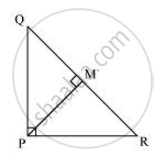Share

# PQR is a triangle right angled at P and M is a point on QR such that PM ⊥ QR. Show that PM^2 = QM . MR - CBSE Class 10 - Mathematics

#### Question

PQR is a triangle right angled at P and M is a point on QR such that PM ⊥ QR. Show that PM2 = QM . MR

#### SolutionLet ∠MPR =  x

In ΔMPR

∠MPR = 180º- 90º - x

∠MRP = 90º - x

Similarity, in ΔMPQ

∠MPQ = 90º - ∠MPR

= 90º - x

∠MQP = 180º - 90º - (90º - x)

∠MQP = x

In ΔQMP and ΔPMR

∠MPQ = ∠MRP

∠PMQ = ∠RMP

∠MQP = ∠MPR

∴ΔQMP ~ ΔPMR (By AAA Similarity criterion)

=>(QM)/(PM) = (MP)/(MR)

=>PM2 = QM x MR

Is there an error in this question or solution?

#### APPEARS IN

NCERT Solution for Mathematics Textbook for Class 10 (2019 to Current)
Chapter 6: Triangles
Ex. 6.50 | Q: 2 | Page no. 150

#### Video TutorialsVIEW ALL 

Solution PQR is a triangle right angled at P and M is a point on QR such that PM ⊥ QR. Show that PM^2 = QM . MR Concept: Pythagoras Theorem.
S